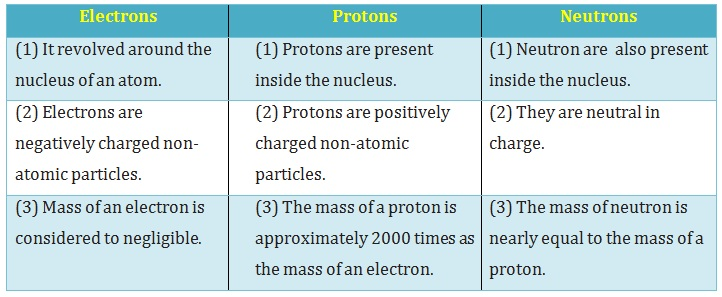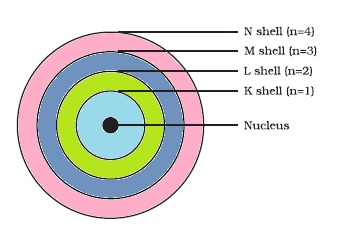Study Materials: ncert solutions

Our ncert solutions for Solutions 4. Structure of The Atom - Text-book Questions | Class 9 Science - Toppers Study is the best material for English Medium students cbse board and other state boards students.

Solutions ⇒ Class 9th ⇒ Science ⇒ 4. Structure of The Atom

# Solutions 4. Structure of The Atom - Text-book Questions | Class 9 Science - Toppers Study

Topper Study classes prepares ncert solutions on practical base problems and comes out with the best result that helps the students and teachers as well as tutors and so many ecademic coaching classes that they need in practical life. Our ncert solutions for Solutions 4. Structure of The Atom - Text-book Questions | Class 9 Science - Toppers Study is the best material for English Medium students cbse board and other state boards students.

## Solutions 4. Structure of The Atom - Text-book Questions | Class 9 Science - Toppers Study

CBSE board students who preparing for class 9 ncert solutions maths and Science solved exercise chapter 4. Structure of The Atom available and this helps in upcoming exams 2023-2024.

### You can Find Science solution Class 9 Chapter 4. Structure of The Atom

• All Chapter review quick revision notes for chapter 4. Structure of The Atom Class 9
• NCERT Solutions And Textual questions Answers Class 9 Science
• Extra NCERT Book questions Answers Class 9 Science
• Importatnt key points with additional Assignment and questions bank solved.

Chapter 4 Science class 9

### Text-book Questions class 9 Science Chapter 4. Structure of The Atom

• Solutions 4. Structure Of The Atom - Text-book Questions | Class 9 Science - Toppers Study
• Class 9 Ncert Solutions
• Solution Chapter 4. Structure Of The Atom Class 9
• Solutions Class 9
• Chapter 4. Structure Of The Atom Text-book Questions Class 9

## Solutions 4. Structure of The Atom - Text-book Questions | Class 9 Science - Toppers Study

Exercises

Q1. Compare the properties of electrons, protons and neutrons.Q2. What are the limitations of J.J. Thomson’s model of the atom?

Thomson’s model explained that atoms are electrically neutral, the results of experiments carried out by other scientists could not be explained by this model.

Q3. What are the limitations of Rutherford’s model of the atom?

Answer: Acording to Rutherford Atomic model :

The electrons revolve around the nucleus. The orbital revolution of the electron is not expected to be stable. Any particle in a circular orbit would undergo acceleration. During acceleration, charged particles would radiate energy. Thus, the revolving electron would lose energy and finally fall into the nucleus. In this case the atom should be highly unstable and hence matter would not exist in the form. But this is not fact we know atom is stable.

Q4. Describe Bohr’s model of the atom.

Answer: Neils Bohr put forward the following postulates about the model of an atom:

(i) Only certain special orbits known as discrete orbits of electrons, are allowed inside the atom.

(ii) While revolving in discrete orbits the electrons do not radiate energy.Q5. Compare all the proposed models of an atom given in this chapter.Q6. Summarise the rules for writing of distribution of electrons in various shells for the first eighteen elements.

The various shells which are also called orbit are represented by the letters K, L, M, N .......

(i) The maximum number of electrons present in a shell is given by theformula 2n2, where ‘n’ is the orbit number like n=1,2,3,4,…. or energy level index, 1,2,3,…. and so on.

For shell K, n = 1

Therefore, maximum number of electrons = 2(1)2 = 2

For shell L, n = 2

Therefore, maximum number of electrons = 2(2)2 = 8

For shell M, n = 3

Therefore, maximum number of electron = 2(3)2 = 18

(ii) The outermost orbit can be accommodated by a maximum number of 8 electrons if they do have more than 8 or 18 electrons.

(iii) Shells are filled with electrons in a step wise manner i.e., the outer shell is not occupied with electrons unless the inner shells are completely filled with electrons.

Q7. Define valency by taking examples of silicon and oxygen.

Answer: The electrons present in the outermost shell of an atom are known as the valence electrons. This number of valence electrons is called valency.

Example of silicon and oxygen

The number of electrons in Silicon = 14

Electronic configuration of Silicon = 2, 8, 4

Therefore, Valance Electrons = 4

Hence Valency = 4,

Example of oxygen

The number of electrons in Oxygen = 8

Electronic configuration = 2, 6

Therefore, Valance electrons = 8 - 6

= 2

Hence Valency of Oxygen = 2

Q8. Explain with examples

(i) Atomic number,

(ii) Mass number,
(iii) Isotopes and

(iv) Isobars. Give any two uses of isotopes.

(i) Atomic Number : The number of protons present in the nucleus of an atom is known as atomic number.

Example : (i) Hydrogen (H) has only 1 proton in its nucleus therefore Atomic Number of Hydrogen is 1.

(ii) Sodium (Na) has 11 protons in its nucleus therefore Atomic Number of Sodium is 11.

(ii) Mass Number : The sum of number of protons and neutrons present in nucleus of an atom is known as atomic mass.

Example : (i) Carbon has 6 protons and 6 neutrons in its nucleus. Therefore Atomic Mass Number of Carbon = 6 + 6 = 12

(iii) Isotopes: The atoms of an element are called isotopes of that element which has equal atomic number but different atomic mass.

Example : Hydrogen Isotopes:(iv) Isobar : The atoms of an element are called isobar of that element which has same atomic mass but different atomic number are called isobar.

Example : Calcium and argon has same mass number of both these elements is 40. But the atomic number of calcium is 20 and argon is 18. These elements are called isobar.

Two Uses of Isotopes are :

(i) An isotope of uranium is used as a fuel in nuclear reactors.

(ii) An isotope of cobalt is used in the treatment of cancer.

Q9. Na+ has completely filled K and L shells. Explain.

Answer : Normally, Electronic configuration of Na is 2, 8, 1.

But when It forms Nathen its configuration is 2, 8

In this case Nacompletely filled K and L shells. As K and L require maximum number of electron that these hold are 2 and 8.

Important Study materials for classes 06, 07, 08,09,10, 11 and 12. Like CBSE Notes, Notes for Science, Notes for maths, Notes for Social Science, Notes for Accountancy, Notes for Economics, Notes for political Science, Noes for History, Notes For Bussiness Study, Physical Educations, Sample Papers, Test Papers, Mock Test Papers, Support Materials and Books.Mathematics Class - 11th

NCERT Maths book for CBSE Students.

books

## Study Materials List:

##### Solutions ⇒ Class 9th ⇒ Science
1. Matter in Our Surroundings
2. Is Matter around us Pure
3. Atoms and Molecules
4. Structure of The Atom
5. The Fundamental Unit of Life
6. Tissues
7. Diversity in Living Organisms
8. Motion
9. Force and Laws of Motion
10. Gravitation
11. Work and Energy
12. Sound
13. Why Do We Fall ill
14. Natural Resources
15. Improvement in Food Resources

New Books